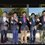# A problem proposal for IPhO 2019 participants

All the best to all the 5 participants will be selected to represent India at IPhO 2019, to be held at Tel Aviv, Israel.

Here is a doable problem for you guys :P

A Dielectric slab of thickness $t$, relative permittivity $\epsilon_r$ is between two fixed metallic parallel plates. Faces of the slab and the plates are parallel. Distance between the plates is $d$. Find the minimum voltage applied between the plates sufficient to rupture the slab, if the breaking stress of slab is $\omega$. Consider a uniform string of length $l$, tension $T$, mass per unit length $\rho$ that is stretched between two immovable walls. Show that the total energy of the string, which is the sum of its kinetic and potential energies $E=\frac {1}{2}\int_0^1 [\rho (\frac {\partial y}{\partial t})+T (\frac {\partial y}{\partial x})^2]dx$. Where $y (x,t)$ is the string's transverse displacement relatively small. The general motion of the string can be represented as a linear superposition of the normal modes: that is $y (x,t)=\sum_{n=1,\infty}(Sin(n\pi\frac {x}{l}))Cos (n\pi\frac {vt}{l}-\phi_n)$. Here $v={\sqrt {\frac{T}{\rho}}}$. Demonstrate that $E=\sum_{n=1,\infty}E_n$, where $E_n=\frac {1}{4}m\omega_n^2A_n^2$ is the energy of $n^{th}$ normal mode. Here, $m=\rho l$ is the mass of the string, and $\omega_n=n\pi\frac {v}{l}$ is the angular frequency of the $n^{th}$ normal place.Note by Pawan Goyal
2 years, 2 months ago

This discussion board is a place to discuss our Daily Challenges and the math and science related to those challenges. Explanations are more than just a solution — they should explain the steps and thinking strategies that you used to obtain the solution. Comments should further the discussion of math and science.

When posting on Brilliant:

• Use the emojis to react to an explanation, whether you're congratulating a job well done , or just really confused .
• Ask specific questions about the challenge or the steps in somebody's explanation. Well-posed questions can add a lot to the discussion, but posting "I don't understand!" doesn't help anyone.
• Try to contribute something new to the discussion, whether it is an extension, generalization or other idea related to the challenge.
• Stay on topic — we're all here to learn more about math and science, not to hear about your favorite get-rich-quick scheme or current world events.

MarkdownAppears as
*italics* or _italics_ italics
**bold** or __bold__ bold
- bulleted- list
• bulleted
• list
1. numbered2. list
1. numbered
2. list
Note: you must add a full line of space before and after lists for them to show up correctly
paragraph 1paragraph 2

paragraph 1

paragraph 2

[example link](https://brilliant.org)example link
> This is a quote
This is a quote
    # I indented these lines
# 4 spaces, and now they show
# up as a code block.

print "hello world"
# I indented these lines
# 4 spaces, and now they show
# up as a code block.

print "hello world"
MathAppears as
Remember to wrap math in $$ ... $$ or $ ... $ to ensure proper formatting.
2 \times 3 $2 \times 3$
2^{34} $2^{34}$
a_{i-1} $a_{i-1}$
\frac{2}{3} $\frac{2}{3}$
\sqrt{2} $\sqrt{2}$
\sum_{i=1}^3 $\sum_{i=1}^3$
\sin \theta $\sin \theta$
\boxed{123} $\boxed{123}$

## Comments

Sort by:

Top Newest

@Archit Boobna @Rajdeep Dhingra best of luck for the TSTs of IPhO 2019.

- 2 years, 1 month ago

Log in to reply

Ya, best of luck. @Pawan Goyal, do you know them? How?

- 2 years, 1 month ago

Log in to reply

first part is too well known and trivial lol

Solution: Kinetic energy: $dK = \frac{1}{2} dm {(\frac{\partial y}{\partial t})}^2 = \frac{1}{2} \rho dx {(\frac{\partial y}{\partial t})}^2 \implies \frac{dK}{dx} = \frac{1}{2} \rho {(\frac{\partial y}{\partial t})}^2$ For potential energy, $dU = T(dl-dx) = \frac{1}{2} T dx {(\frac{\partial y}{\partial x})}^{2} \implies \frac{dU}{dx} = \frac{1}{2} \rho v^2 {(\frac{\partial y}{\partial x})}^{2}$ Adding the two, we get $\frac{dE}{dx} = \frac{1}{2} (\rho(\frac {\partial y}{\partial t})^2+T(\frac{\partial y}{\partial x})^2)$ and so $\boxed{E = \int_{x=0}^{L}{\frac{1}{2} (\rho(\frac {\partial y}{\partial t})^2+T(\frac{\partial y}{\partial x})^2)}dx}$This is in general true for any one-dimensional linear wave, transverse or longitudinal, propagating or non-propagating.

And for the second part

Let $y(x,t)=\sum_{n=1,\infty}y_n(x, t)$. Plug $\frac{\partial y}{\partial x}$ and $\frac{\partial y}{\partial t}$ in the energy equation. You should be able to show that $\int_{x=0}^{L}{\frac{\partial y_a}{\partial x} \cdot \frac{\partial y_b}{\partial x}}\ dx = 0$ if $a \neq b$ and likewise for $t$. Then, the only terms that remain are the squared terms, and the answer is immediate.

Overall this question is quite easy and almost everybody would get a full score in this one.

- 1 year, 4 months ago

Log in to reply

×

Problem Loading...

Note Loading...

Set Loading...